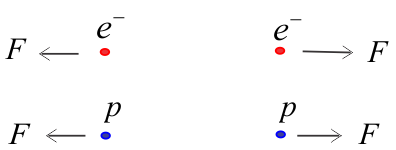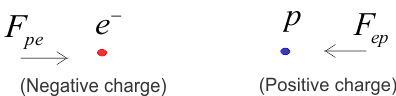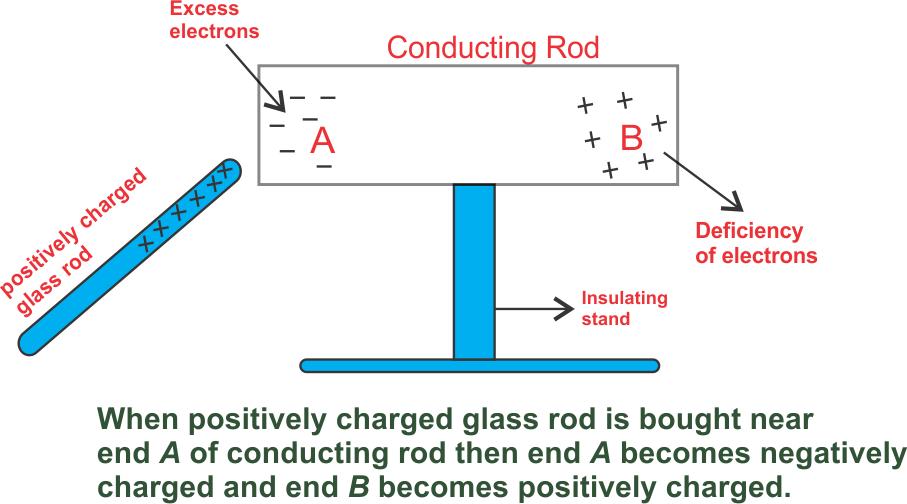# Electrostatics : Electric Charge, Basic properties of electric charge

• Electric Charge
• Methods of charging
• Basic properties of electric charge

In basic properties of electric charge, we will learn that charge is conserved. We will also learn about quantization of charge and additivity of charges.

This chapter lays a foundation for concepts like electric current and magnetism. I would tell you to put extra effort into learning these concepts. These concepts comes in handy while studying electricity and magnetism.

## Electric Charge

Electric charge is a fundamental property associated with elementary particles. It accompanies fundamental particles whenever that exists. Electron, proton, neutrons are a few examples of fundamental particles.

According to William Gilbert,

The charge is something possessed by material objects that make it possible for them to exert electrical forces and to respond to electrical force.

We know that in an atom electrons revolve around a nucleus which has a positive charge. Electric charge is the property responsible for electric forces acting between nucleus and electrons in an atom. This electric force between the nucleus and electrons bind the atom together.
Charges are of two kinds
(i) negative charge
(ii) positive charge
In an atom electron are particles having a negative charge? The nucleus consists of protons and neutrons. In a nucleus of an atom, protons have a positive charge and neutrons are neutral. The experiments lead to the fundamental results that

(1) like charges repel,(2) unlike charges attractThe electric force between two electrons is the same as the electric force between two protons kept at the same distance apart. That is both sets repel each other.
The electric force between an electron and proton placed at the same distance apart is not repulsive but attractive in nature.
Assignment of a negative charge on the electron and a positive charge on a proton is only a convention. It does not mean that charge on an electron is less than that on the proton. Importance of electric forces is that it encompasses almost every field associated with our life. It is important because all matter around us consists of atoms or molecules in which electric charges are exactly balanced.

### Unit of charge

• The charge on a system can be measured by comparing it with the charge on a standard body.
• SI unit of charge is Coulomb written as C.
• Coulomb is the charge flowing through the wire in 1 second if the electric current in it is 1A.
• Charge on electron is $e=-1.602 \times 10^{ -19} C$ and charge on proton is positive of this value.

## Conductors and Insulators

There is a category of materials in which electric charges can flow easily while in other materials charges cannot flow easily. Substances through which electric charges can flow easily are called conductors. All metals like copper, aluminium etc. are good conductors of electricity.

Substances through which electric charges cannot flow are called insulators. Few examples of insulating materials are glass, rubber, mica, plastic, dry wood etc.

Presence or absence of free electrons in a material makes it a conductor or insulator. Conductors have free electrons which are loosely held by nuclei of their atoms. Insulators do not have free electrons. In insulators, electrons are strongly held by nuclei of their atoms.

It is important to note that

1. The charge transferred to a conductor gets distributed over the entire surface.

2. The charge transferred to an insulator stays at the same place.

Semiconductors are the third class of materials. Electrical properties of semiconductors are somewhat between insulators and conductors. Silicon and germanium are examples of semiconductors.

Question 1. Why do metals conduct electricity?

Answer. Atoms of metals have outer electrons which are not tied to any one atom. These electrons can move freely within the structure of a metal when an electric current is applied. That is why metals conduct electricity.

### What happens when a body is charged?

Case 1: Body has a positive charge
When the body has a positive charge, it means electrons are somehow removed from the body. This results also in a decrease of mass of the body. The decrease in the mass of the body equals the total mass of electrons removed from the body.

Case 2: Body has a negative charge
When the body has a negative charge, it means electrons are somehow added to the body. This results in an increase of mass of the body. Increase in the mass of the body equals the total mass of electrons added to the body.

## Methods of charging

There are three main methods for charging of a body

### (i) Charging by rubbing and Fractional electricity:-

Rubbing as the term suggest is moving two things back and forth against each other. The simplest way to experience electric charge is to rub certain bodies among each other. Rubbing or friction makes electrons move. This gives one material a positive charge and the other a negative charge. The charges stay on the surfaces of the materials until they can flow or they discharge.
If we pass a comb through hairs, comb becomes charged and can attract small pieces of paper. This is because the comb might have lost its electrons or acquired some electrons when we rub it with hairs. Now, this comb is a charged body. The net charge on the comb interacts with the net charge on small pieces of paper which results in attraction. Many such solid materials are known which on rubbing attract light objects like a light feather, bits of papers, straw etc.

Positive charge Negative charge
Glass rod Silk Cloth
Woolen cloth or cat skin Amber, ebonite, rubber rod
Woolen carpet Rubber shoe soles
Woolen coat Plastic seat

Explanation of appearance of electric charge on rubbing is simple. Material bodies consist of a large number of electrons and protons in equal number and hence is in neutral in their normal state. But when a glass rod is rubbed with a silk cloth, electrons are transferred from glass rod to silk cloth. The glass rod becomes positively charged and the silk cloth becomes negatively charged as it receives extra electrons from the glass rod. In this case rod after rubbing, comb after passing through dry hairs becomes electrified and these are the example of frictional electricity.

### (ii) Charging by induction (Electrostatic Induction):-

The temporary electrification of a conductor, when a charged body is brought near it is called electrostatic induction.Electrostatic Induction :- Electrical charges in the conductor are redistributed When a body is charged this way there is no transfer of electrons from one body to other. This happens because there is no physical contact taking place between charging body and conductor being charged.
If a charged body is brought near an uncharged body, then the neutral body becomes oppositely charged. By induction method, we can charge any type of material body.

For further reading you can visit this very good article
https://www.school-for-champions.com/science/static_induction.htm

### (iii) By conduction (by touch without rubbing):-

Because of having excess free electrons in metals they can be charged by conduction. When we bring two conductors, one charged and other uncharged in contact, the same type of charge will appear on both the conductors.

## Basic properties of electric charge

Charges adds up like real numbers i. e., they are Scalars more clearly if any system has n number of charges $q_1$, $q_2$, $q_3$, qn then total charge of the system is $q = q_1 + q+2 + q_3 + ................ q_n$.
Proper sign have to be used while adding the charges for example if
$q_1 = +1C$, $q_2 = -2C$ and $q_3 = +4C$
then total charge of the system is
$q = q_1 + q_2 + q_3$
or
$q = (+1) + (-2) + (+4) C = (+3) C$

### (ii) conservation of charge :

• Charge of an isolated system is conserved.
• This means net charge of a system is always constant.
• Charge can not be created or destroyed but charged particles can be transferred from one body to another.

### (iii) Quantization of charge

• fundamental charge is the magnitude of charge on an electron which is $e=1.6\times 10^{-19}C$
• charge Q on a body is always denoted by
$Q = \pm ne$ where
n = any integer positive or negative
• Charged body always attain charge in multiples of $e$ not in fractions.
• This is called *Quantization of charge* where it varies in discrete steps.

### Solved Example

These are some solved examples related to electric charge and properties of electric charge given in this page.
Question 1 Is there any transfer of mass when electrons are transferred from one substance to another?

Question 2 (NCERT) A polythene piece rubbed with wool is found to have a negative charge of 3.2 × 10–7 C.
(a) Estimate the number of electrons transferred (from which to which?)
(b) Is there a transfer of mass from wool to polythene?

Question 3 When a glass rod is rubbed with a silk cloth, charges appear on both. A similar phenomenon is observed with many other pairs of bodies. Explain how this observation is consistent with the law of conservation of charge.

Question 4 (a) Explain the meaning of the statement ‘electric charge of a body is quantized’.
(b) Why can one ignore quantization of electric charge when dealing with macroscopic i.e., large scale charge?

### Problem solving tips

• Firstly read your problem carefully. Reading and understanding the problem is the first step towards solving it.
• Once you have read and understood the problem then try to analyze it using your previous knowledge of the topic.
• Comprehensive knowledge of the topic is required and it is prerequisite for solving any problem.
• Now look for the known and unknown quantities.
• Try to find the formula that can be applied to the problem based on your known and unknown quantities.
• Now apply the formula, perform the desired calculation and obtain the results.
• Try to interpret your results.